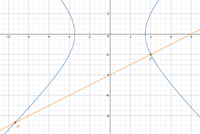# Cartesian eq- help with this q pls ??

#### pka

##### Elite Member
View attachment 17489
really struggling with part b and c
part A I calculated t= +/- 3
Can you find both $$\dfrac{dx}{dt}~\&~\dfrac{dy}{dt}~?$$ If yes then $$\dfrac{dx}{dy}=\dfrac{dx/dt}{dy/dt}$$.
If no what have you been doing in the course?

•topsquark

#### MarkFL

##### Super Moderator
Staff member
Hello, and welcome to FMH!For part (b) consider:

$$\displaystyle x^2=t^2+6+\frac{9}{t^2}$$

$$\displaystyle y^2=t^2-6+\frac{9}{t^2}$$

What do you get upon subtracting the latter from the former?

•topsquark

#### Oors

##### New member
Hello, and welcome to FMH!For part (b) consider:

$$\displaystyle x^2=t^2+6+\frac{9}{t^2}$$

$$\displaystyle y^2=t^2-6+\frac{9}{t^2}$$

What do you get upon subtracting the latter from the former?
Thank you I got the answer !

#### Oors

##### New member
Hello, and welcome to FMH!For part (b) consider:

$$\displaystyle x^2=t^2+6+\frac{9}{t^2}$$

$$\displaystyle y^2=t^2-6+\frac{9}{t^2}$$

What do you get upon subtracting the latter from the former?
How do you obtain an answer for part c ??#### Jomo

##### Elite Member
Find the slope at t=1. Then what would the slope of the normal line be?

#### Oors

##### New member
Find the slope at t=1. Then what would the slope of the normal line be?
got answer y+2=1/2(x-4) for the equation of the line however I am stuck on how to do part (ii)

#### MarkFL

##### Super Moderator
Staff member
Okay, for part (b) you should have found that:

$$\displaystyle x^2-y^2=12$$

The slope of the normal lime is:

$$\displaystyle m=\left.-\frac{dx}{dy}\right|_{t=1}=\frac{1}{2}$$

And so the normal line to $$P$$ is:

$$\displaystyle y=\frac{1}{2}(x-4)-2=\frac{1}{2}x-4$$

This is equivalent to your result. To find the other point of intersection, we can substitute for $$y$$:

$$\displaystyle x^2-\left(\frac{1}{2}x-4\right)^2=12$$

What do you get when you solve the above for $$x$$?